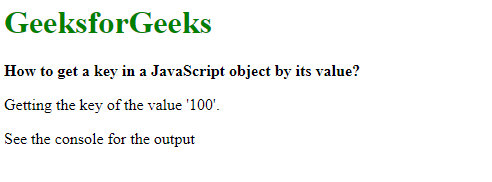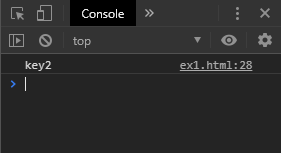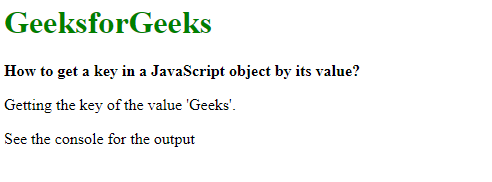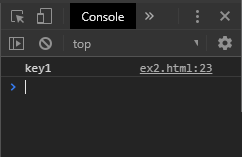# How to get a key in a JavaScript object by its value ?

Method 1: Checking all the object properties to find the value: The values of the object can be found by iterating through its properties. Each of these properties con be checked to see if they match the value provided. The properties of the object are obtained by using a for loop on the object. These properties are then checked with the object’s hasOwnProperty() method to make sure it is a direct property of the object and not an inherited one.
Each property is then checked if they are equal to the value to be found. If the value matches, then the property is returned. This is the key to the value of the object.

Example:

 ` ` `<``html``> ` ` `  `<``head``> ` `    ``<``title``> ` `        ``How to get a key in a JavaScript ` `        ``object by its value ? ` `    `` ` ` ` ` `  `<``body``> ` `    ``<``h1` `style``=``"color: green"``>GeeksforGeeks ` `     `  `    ``<``b``> ` `        ``How to get a key in a JavaScript ` `        ``object by its value ? ` `    `` ` `     `  `    ``<``p``>Getting the key of the value '100'. ` `     `  `    ``<``p``>See the console for the output ` ` `  `    ``<``script``> ` `        ``function getKeyByValue(object, value) { ` `            ``for (var prop in object) { ` `                ``if (object.hasOwnProperty(prop)) { ` `                    ``if (object[prop] === value) ` `                    ``return prop; ` `                ``} ` `            ``} ` `        ``} ` `     `  `        ``var exampleObject = { ` `            ``key1: 'Geeks', ` `            ``key2: 100, ` `            ``key3: 'Javascript' ` `        ``}; ` ` `  `        ``ans = getKeyByValue(exampleObject, 100); ` `         `  `        ``console.log(ans); ` `    `` ` ` ` ` `  ` `

Output:Console Output:Method 2: Using the find method() to compare the keys: The Object.keys() method is used to return all the keys of the object. On this array of keys, the find() method is used to test if any of these keys match the value provided. The find() method is used to return the value of the first element that satisfies the testing function. If the value matches, then this condition is satisfied and the respective key is returned. This is the key to the value of the object.

Note: This method was added in the ES6 specification and may not be supported on older browser versions.

Syntax:

 `function` `getKeyByValue(object, value) { ` `    ``return` `Object.keys(object).find(key => object[key] === value); ` `} `

Example:

 ` ` `<``html``> ` `     `  `<``head``> ` `    ``<``title``> ` `        ``How to get a key in a JavaScript ` `        ``object by its value ? ` `    `` ` ` ` ` `  `<``body``> ` `    ``<``h1` `style``=``"color: green"``>GeeksforGeeks ` `     `  `    ``<``b``>How to get a key in a JavaScript object by its value? ` `     `  `    ``<``p``>Getting the key of the value 'Geeks'. ` `     `  `    ``<``p``>See the console for the output ` ` `  `    ``<``script``> ` `        ``function getKeyByValue(object, value) { ` `            ``return Object.keys(object).find(key =>  ` `                    ``object[key] === value); ` `        ``} ` `     `  `        ``var exampleObject = { ` `            ``key1: 'Geeks', ` `            ``key2: 100, ` `            ``key3: 'Javascript' ` `        ``}; ` `     `  `        ``ans = getKeyByValue(exampleObject, 'Geeks'); ` `        ``console.log(ans); ` `    `` ` ` ` ` `  ` `

Output:Console Output:My Personal Notes arrow_drop_upCheck out this Author's contributed articles.

If you like GeeksforGeeks and would like to contribute, you can also write an article using contribute.geeksforgeeks.org or mail your article to contribute@geeksforgeeks.org. See your article appearing on the GeeksforGeeks main page and help other Geeks.

Please Improve this article if you find anything incorrect by clicking on the "Improve Article" button below.